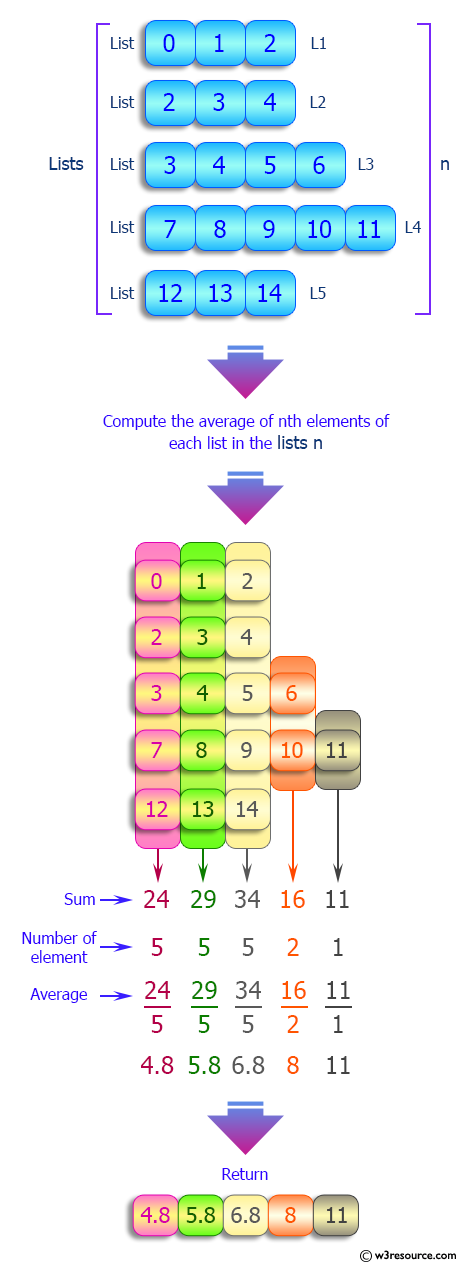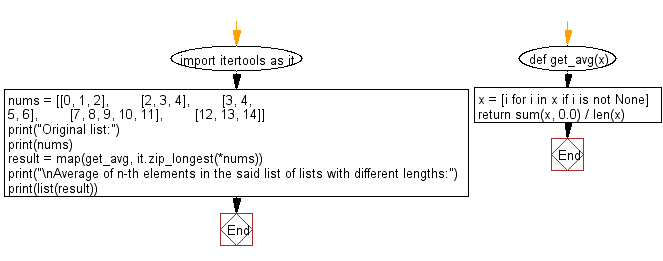﻿ Python: Compute the average of n-th elements in a given list of lists with different lengths - w3resource# Python: Compute the average of nth elements in a given list of lists with different lengths

## Python List: Exercise - 197 with Solution

Write a Python program to compute the average of nth elements in a given list of lists with different lengths.

Sample Solution:

Python Code:

``````import itertools as it
nums = [[0, 1, 2],
[2, 3, 4],
[3, 4, 5, 6],
[7, 8, 9, 10, 11],
[12, 13, 14]]

print("Original list:")
print(nums)
def get_avg(x):
x = [i for i in x if i is not None]
return sum(x, 0.0) / len(x)
result = map(get_avg, it.zip_longest(*nums))
print("\nAverage of n-th elements in the said list of lists with different lengths:")
print(list(result))
```
```

Sample Output:

```Original list:
[[0, 1, 2], [2, 3, 4], [3, 4, 5, 6], [7, 8, 9, 10, 11], [12, 13, 14]]

Average of n-th elements in the said list of lists with different lengths:
[4.8, 5.8, 6.8, 8.0, 11.0]
```

Pictorial Presentation:Flowchart:## Visualize Python code execution:

The following tool visualize what the computer is doing step-by-step as it executes the said program:

Python Code Editor:

Have another way to solve this solution? Contribute your code (and comments) through Disqus.

What is the difficulty level of this exercise?

Test your Python skills with w3resource's quiz

﻿

## Python: Tips of the Day

Floor Division:

When we speak of division we normally mean (/) float division operator, this will give a precise result in float format with decimals.

For a rounded integer result there is (//) floor division operator in Python. Floor division will only give integer results that are round numbers.

```print(1000 // 300)
print(1000 / 300)```

Output:

```3
3.3333333333333335```